## 1. Introduction

The best-first search is a class of search algorithms aiming to find the shortest path from a given starting node to a goal node in a graph using heuristics. The AO* algorithm is an example of the best-first search class. In this tutorial, we’ll discuss how it works.

This class should not be mistaken for breadth-first search, which is just an algorithm and not a class of algorithms. Moreover, the best-first search algorithms are informed, which means they have prior knowledge about the distances from heuristics. However, the breadth-first search algorithm is uninformed.

## 2. AO* Algorithm

The AO* algorithm is based on AND-OR graphs to break complex problems into smaller ones and then solve them. The AND side of the graph represents those tasks that need to be done with each other to reach the goal, while the OR side stands alone for a single task. Let’s see an example below: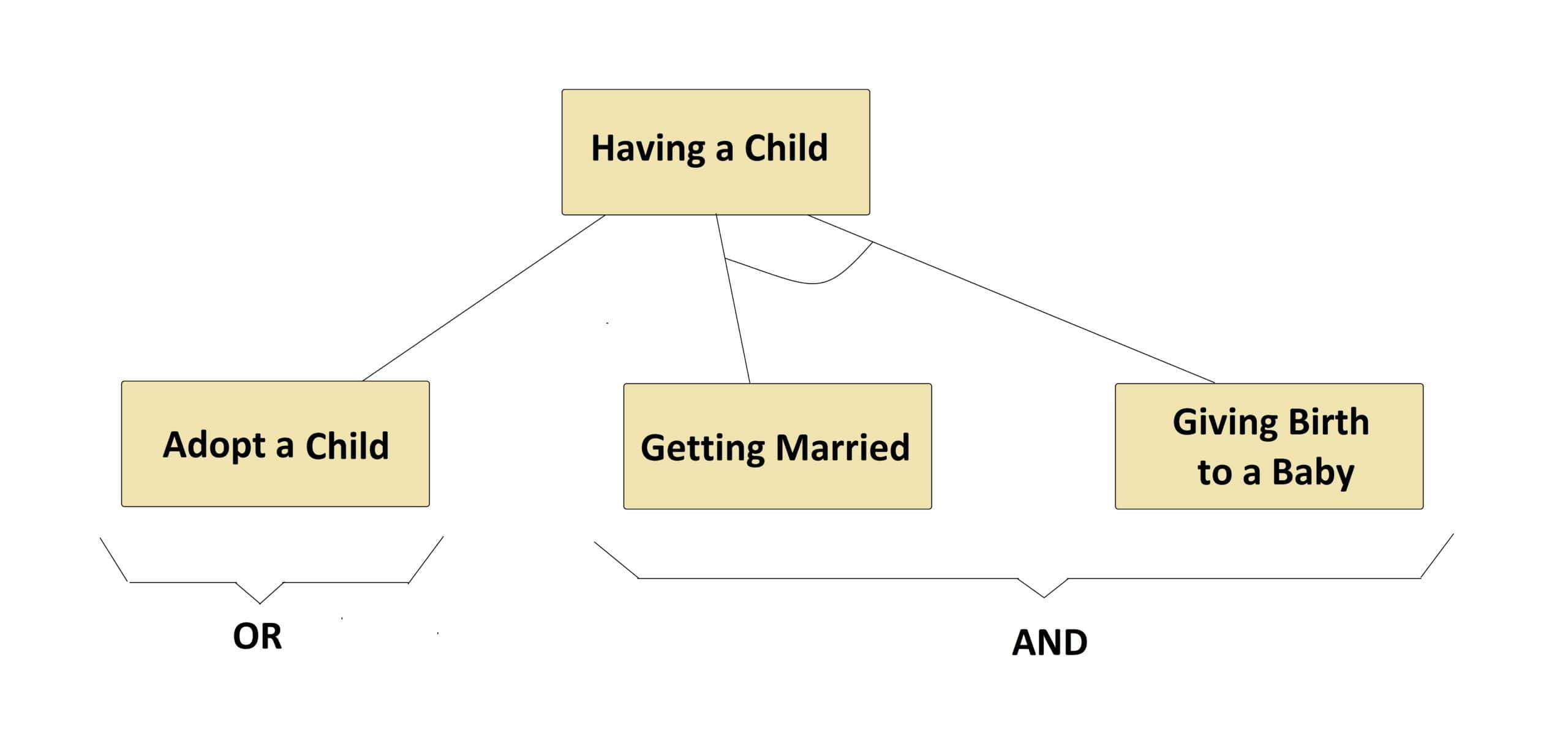In the above example, we can see both tasks of the AND side, which are connected by an AND-ARCS, need to be done to have a child, while the OR side lets having a child just by a single task of adoption.

In general, in a graph for each node, there could be multiple OR and AND sides, where each AND side itself may have multiple successor nodes connected to each other by an AND-ARCS.

## 3. How Does AO* Work?

AO* works based on this formula:, whereis the actual cost of going from the starting node to the current node,is the estimated or heuristic cost of going from the current node to the goal node, andis the actual cost of going from the starting node to the goal node.

Let’s see an example below to explain AO* step by step to find the lowest cost path from the starting nodeto the goal node: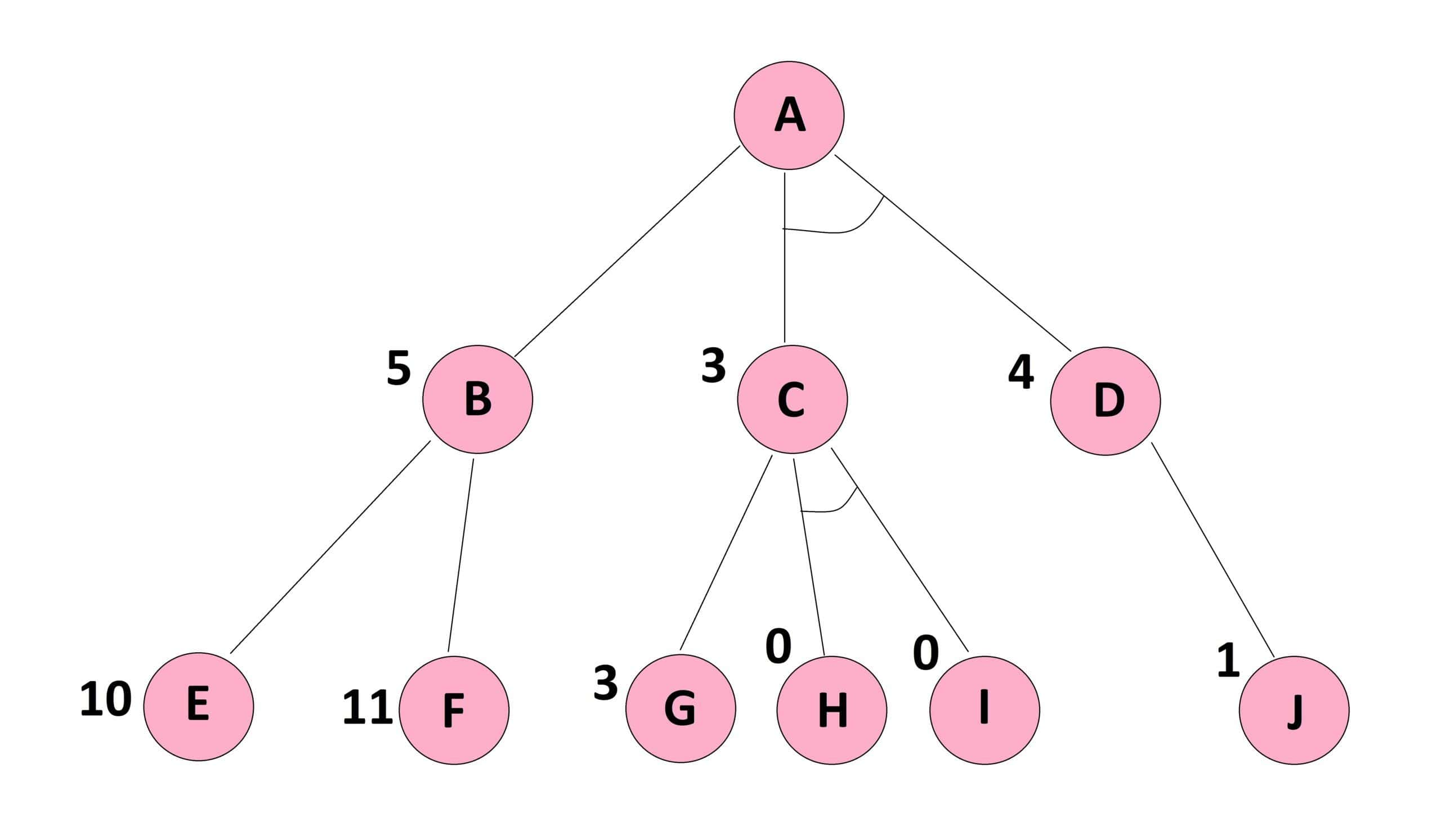It should be noted that the cost of each edge is the same as, and the heuristic cost to reach the goal node from each node of the graph is shown beside it.

Moreover, it is worth mentioning that we may not see the goal node in this graph, but it does not matter because we already know the heuristic costs from each node of this graph to that unseen goal node.

### 3.1. Forward Propagation

First, we begin from nodeand calculate each of the OR side and AND side paths. The OR side path, whereis the cost of the edge betweenand, andis the estimated cost fromto the goal node.

The AND side path, where the firstis the cost of the edge betweenand,is the estimated cost fromto the goal node, the secondis the cost of the edge betweenand, andis the estimated cost fromto the goal node. Let’s see an example below: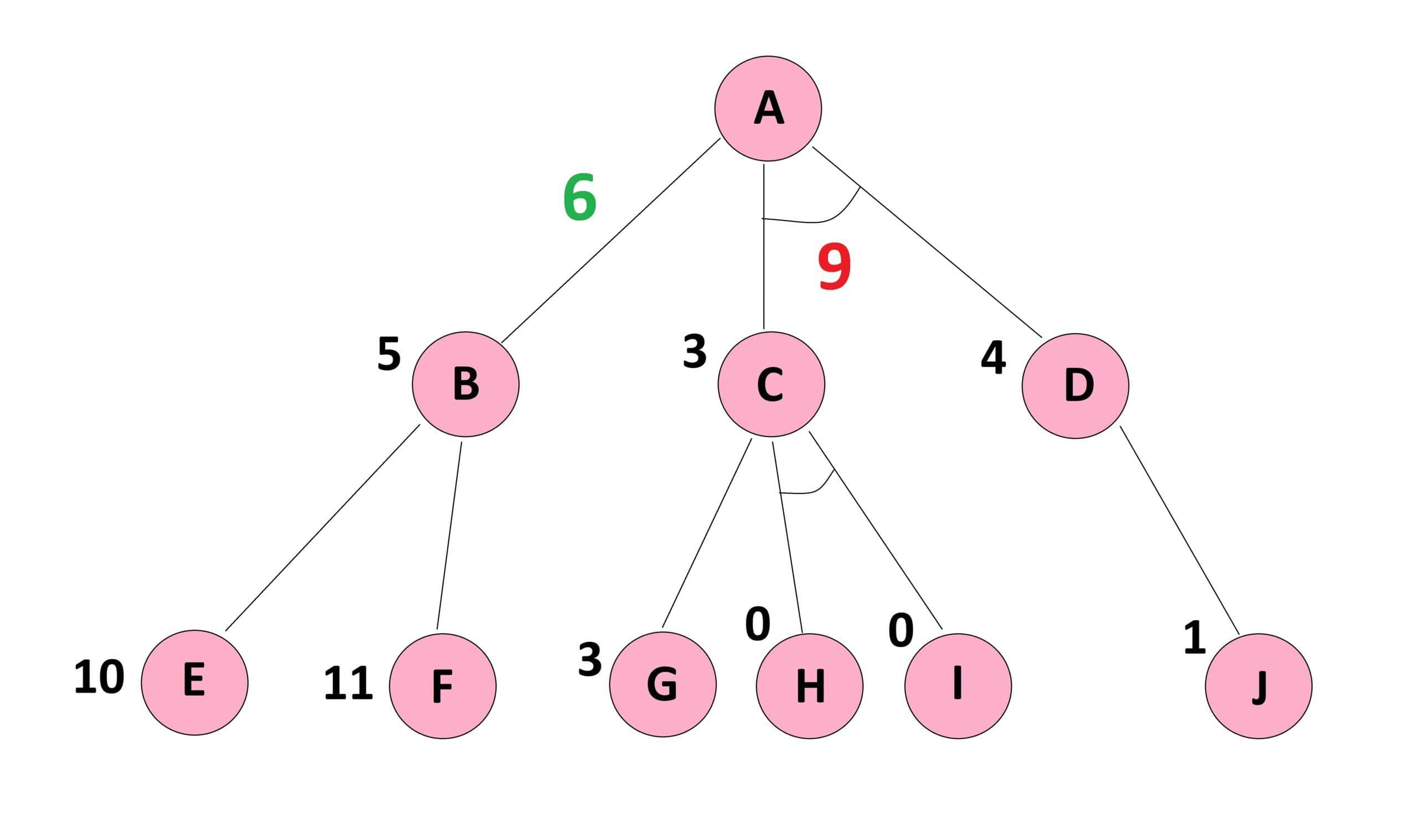Since the cost ofis the minimum cost path, we proceed on this path in the next step.

Here, someone may ask why we do not stop here since we have already found the minimum cost path fromto the goal node. The answer is that such a path may not be the correct minimum cost path because we have made our calculations based on the heuristics down to only one level. However, the given graph has provided us with a deeper level whose calculations may update the achieved values.

### 3.2. Reaching the Last Level and Back Propagation

In this step we continue on thefromto its successor nodes i.e.,and, whereand. Here,has a lower cost and would be chosen.

Now, we have reached the bottom of the graph where no more level is given to add to our information. Therefore, we can do the backpropagation and correct the heuristics of upper levels. In this vein, the updated, and as a consequence the updated. Let’s see an example below: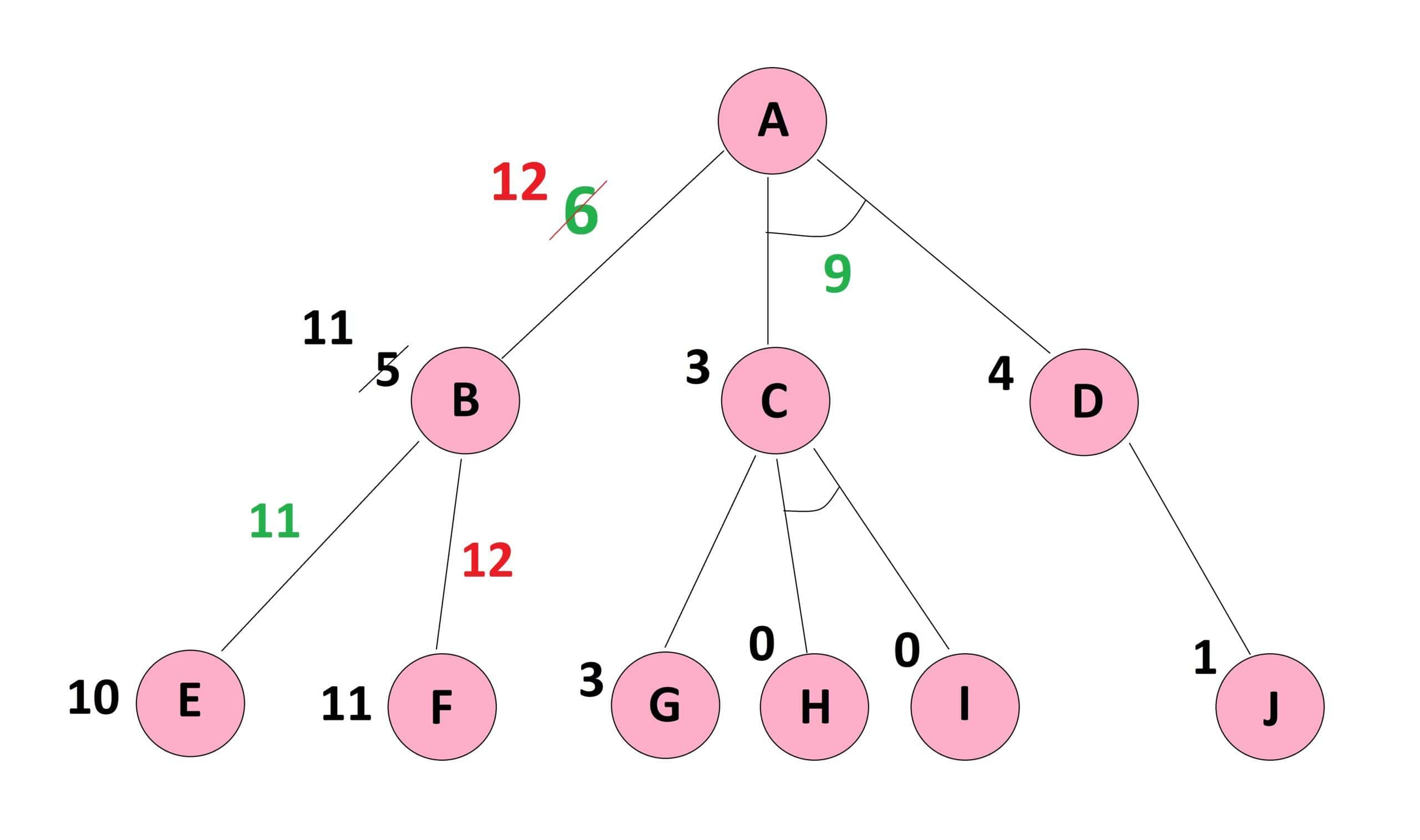Now, we can see thatwith a cost ofis lower than the updatedwith a cost of. Therefore, we need to proceed on this path to find the minimum cost path fromto the goal node.

It is worth mentioning that if the updatedhad a lower cost than, then we were done, and no more calculations would be required.

### 3.3. Correcting the Path from Start Node

In this step, we do the calculations for the AND side path, i.e.,, and first explore the paths attached to node. In this node again we have an OR side where, and an AND side where, and as a consequence the updated.

Also, the updated, since. By these updated values forand, the updated. Let’s see an example below: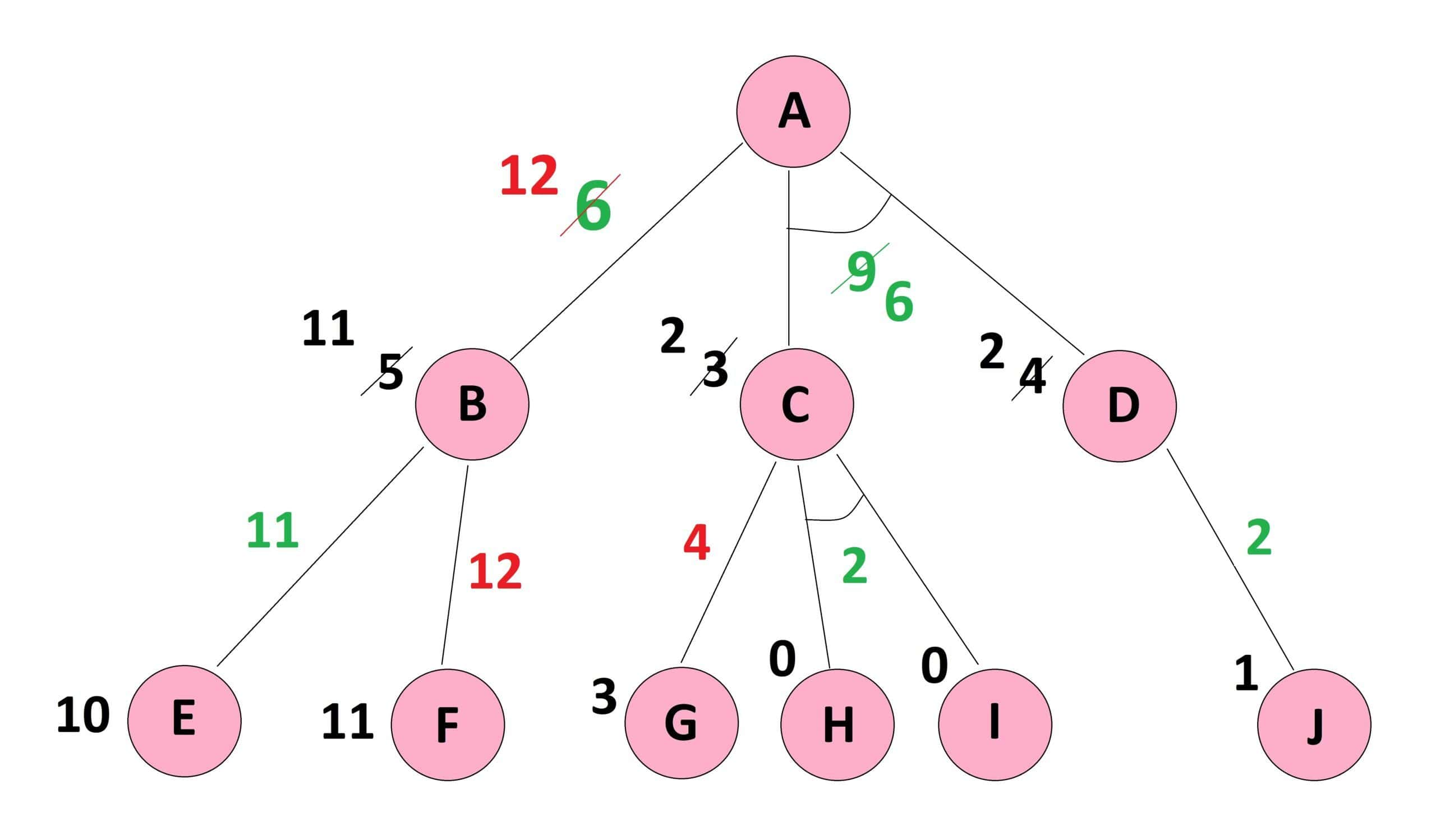This updatedwith the cost ofis still less than the updatedwith the cost of, and therefore, the minimum cost path fromto the goal node goes fromby the cost of. We are done.

## 4. Pseudocode

In this section, we present the pseudocode of the AO* algorithm. As we have seen in the above example, the process goes into a cycle of forward and backward propagation until there is no more change in the minimum cost path. To simplify, we did not go into details of the backpropagation since it follows the same rules of forward propagation but in the opposite direction. Let’s see the pseudocode below:## 5. AO* Performance

The AO* algorithm is not optimal because it stops as soon as it finds a solution and does not explore all the paths. For example, if the early heuristic value ofwere more than, we would never explore this side, and the solution remained for the OR side.

However, AO* is complete, meaning it finds a solution, if there is any, and does not fall into an infinite loop. Moreover, the AND feature in this algorithm reduces the demand for memory.

The space complexity comes in polynomial order, while the time complexity is, wherestands for branching andis the maximum depth or number of levels in the search tree.

## 6. Conclusion

In this tutorial, we examined AO* as a sample of best-first search algorithms. We noticed that the strength of this algorithm comes from a divide and conquer strategy. The AND feature brings all the tasks of the same goal under one umbrella and reduces the complexities. However, such a benefit comes at the cost of losing optimality.